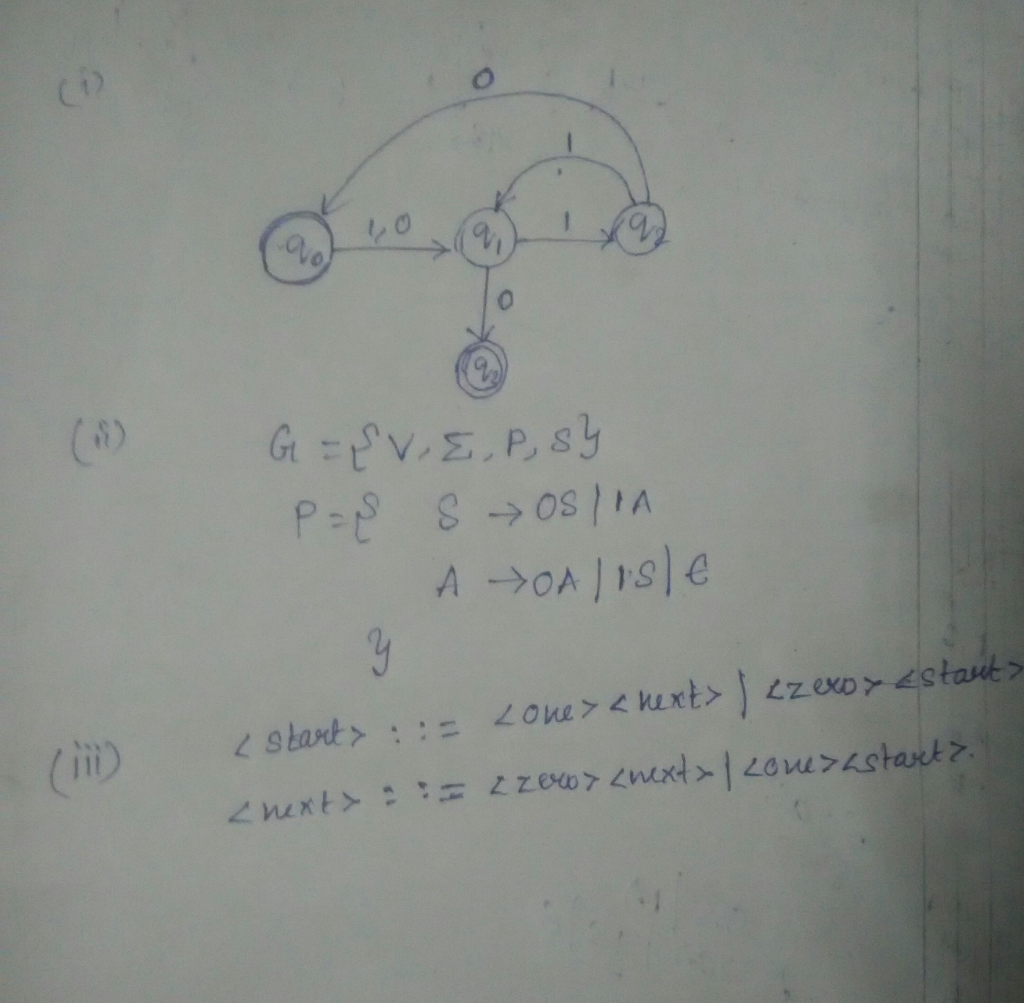# Answered! Discrete Math L is a language that consists of all the binary strings with an odd number of…

Discrete Math

L is a language that consists of all the binary strings with an odd number of
digit “1”.

Don't use plagiarized sources. Get Your Custom Essay on
Answered! Discrete Math L is a language that consists of all the binary strings with an odd number of…
GET AN ESSAY WRITTEN FOR YOU FROM AS LOW AS \$13/PAGE

(I)      Write the simplest grammar for L in a Finite State Machine format.Explain how you get your answer.

(ii)     Write the simplest grammar for L in a Phase Structure Grammar format. Explain how you get your answer.

(iii)    Write the simplest grammar for L in a BNF notation.

1)

Finite state machine that accepts odd number of 1’s

Let us consider q0 as initial state and when the is zero or one it moves to state q1.

Here q3 is the accepting state.

For example consider,

the string 1110.